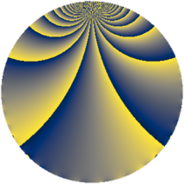# Properties

 Label 1694.2.mLevel $1694$ Weight $2$ Character orbit 1694.m Rep. character $\chi_{1694}(155,\cdot)$ Character field $\Q(\zeta_{11})$ Dimension $660$ Sturm bound $528$

# Related objects

## Defining parameters

 Level: $$N$$ $$=$$ $$1694 = 2 \cdot 7 \cdot 11^{2}$$ Weight: $$k$$ $$=$$ $$2$$ Character orbit: $$[\chi]$$ $$=$$ 1694.m (of order $$11$$ and degree $$10$$) Character conductor: $$\operatorname{cond}(\chi)$$ $$=$$ $$121$$ Character field: $$\Q(\zeta_{11})$$ Sturm bound: $$528$$

## Dimensions

The following table gives the dimensions of various subspaces of $$M_{2}(1694, [\chi])$$.

Total New Old
Modular forms 2680 660 2020
Cusp forms 2600 660 1940
Eisenstein series 80 0 80

## Trace form

 $$660q + 2q^{2} + 4q^{3} - 66q^{4} + 4q^{6} + 2q^{8} + 672q^{9} + O(q^{10})$$ $$660q + 2q^{2} + 4q^{3} - 66q^{4} + 4q^{6} + 2q^{8} + 672q^{9} + 10q^{11} - 18q^{12} - 108q^{13} - 20q^{15} - 66q^{16} + 12q^{17} + 10q^{18} + 28q^{19} + 12q^{21} - 34q^{22} + 8q^{23} + 4q^{24} - 42q^{25} + 40q^{27} + 44q^{29} + 40q^{30} - 48q^{31} + 2q^{32} + 44q^{33} + 28q^{34} + 4q^{35} - 54q^{36} + 24q^{37} - 104q^{38} + 56q^{39} + 12q^{41} + 48q^{43} + 10q^{44} + 24q^{45} + 32q^{46} + 48q^{47} + 4q^{48} - 66q^{49} + 30q^{50} - 126q^{51} + 24q^{52} + 32q^{53} + 64q^{54} - 28q^{55} + 58q^{57} - 72q^{58} + 60q^{59} + 24q^{60} + 64q^{61} + 16q^{62} - 66q^{64} - 44q^{65} + 26q^{66} - 48q^{67} + 12q^{68} + 72q^{69} - 36q^{70} - 48q^{71} + 10q^{72} - 20q^{73} + 52q^{74} + 100q^{75} - 38q^{76} + 8q^{77} + 64q^{78} + 4q^{79} + 740q^{81} - 44q^{82} + 100q^{83} + 12q^{84} - 108q^{85} + 44q^{86} + 72q^{87} - 56q^{88} + 60q^{89} - 104q^{90} - 108q^{91} + 8q^{92} + 120q^{93} - 144q^{94} - 88q^{95} + 4q^{96} + 84q^{97} + 2q^{98} + 130q^{99} + O(q^{100})$$

## Decomposition of $$S_{2}^{\mathrm{new}}(1694, [\chi])$$ into newform subspaces

The newforms in this space have not yet been added to the LMFDB.

## Decomposition of $$S_{2}^{\mathrm{old}}(1694, [\chi])$$ into lower level spaces

$$S_{2}^{\mathrm{old}}(1694, [\chi]) \cong$$ $$S_{2}^{\mathrm{new}}(121, [\chi])$$$$^{\oplus 4}$$$$\oplus$$$$S_{2}^{\mathrm{new}}(242, [\chi])$$$$^{\oplus 2}$$$$\oplus$$$$S_{2}^{\mathrm{new}}(847, [\chi])$$$$^{\oplus 2}$$